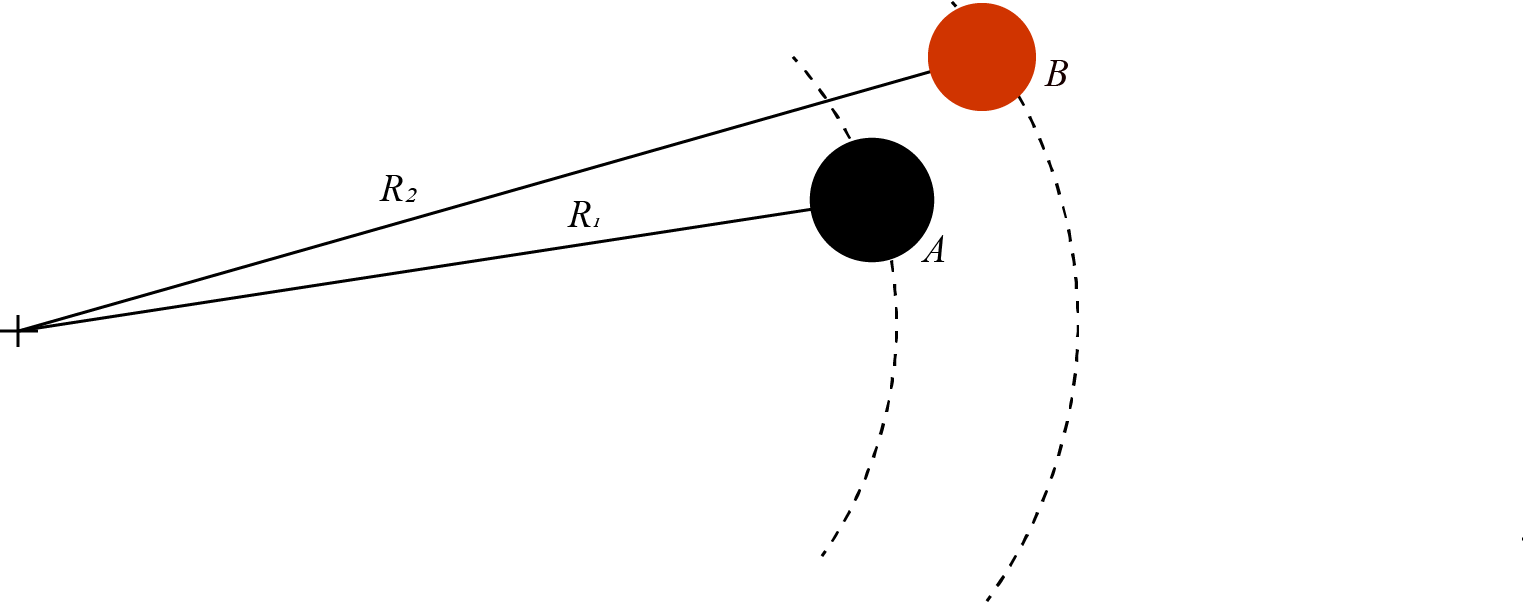# Yoyo Spin#

A performer spins two yoyos at full length in a loop as part of a trick. Yoyo A has a string length $$R\_{1} = {{params.r1}}cm$$ and yoyo B has a string length $$R\_{2} = {{params.r2}}cm$$. What is the maximum speed of each yoyo if the strength of the string restricts centripetal acceleration to $$g$$ (Earth’s gravitational constant)?## Part 1#

Determine the maximum speed for yoyo A.

Please enter in a numeric value in $$m/s$$.

## Part 2#

Determine the maximum speed for yoyo B.

Please enter in a numeric value in $$m/s$$.